# [Improving Deep Neural Networks] week3. Hyperparameter tuning, Batch Normalization and Programming Frameworks

[TOC]

## Hyperparameter parameters

Tips for hyperparam-tuning.

### Tuning process

Many hyperparams to tune, mark importance by colors (red > yellow > purple):How to select set of values to explore ?

• Do NOT use grid search (grid of n * n)

— this was OK in pre-DL era.

• try random values.

reason: difficule to know which hyperparam is most important, by randomization, can try out nn distinct values for each hyperparam.*
In extreme case, one is `alpha`, the other is `epislon`.

• in grid search: only n distinct values of alpha are tried
• in random choice: can have n*n distinct values of alpha• Coarse to fine sample scheme: zoom in to smaller regions of hyperparam space and re-sample more densely.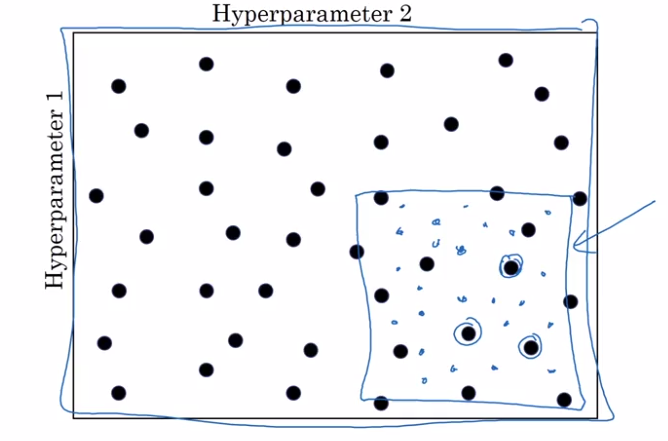### Using an appropriate scale to pick hyperparameters

"Sampling at random", but at appropriate scale, not uniformly.
example: choice of alpha in [0.001, 1]
sample uniformly at log scale is more resonable: equal resources are used to search at each scale.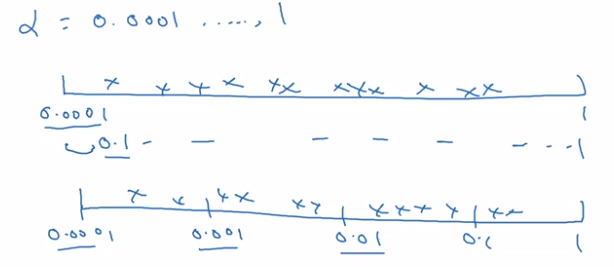implementation:

```r = -4 * np.random.rand()  # -4 <= r <= 0, uniformly at randome
alpha = np.exp(10, r) # 10e-4 <= alpha <= 1.0
```

sampling beta for exp-weighted-avg: sample in the range of [0.9, 0.999]
→ convert to sampling 1-beta, which is in range [0.0001, 0.1]

### Hyperparameters tuning in practice: Pandas vs. Caviar

Tricks on how to organize hyper-param-tuning process.

re-test hyperparams occasionally: intuitions get stale, re-evaluate hyperparams every several months.

Two major schools of training

• Panda approach: babysitting one model

Huge dataset, limited computing resources, can only train one model → babysit the model as it's training. Watch learning curve, try changing hyparams once a day.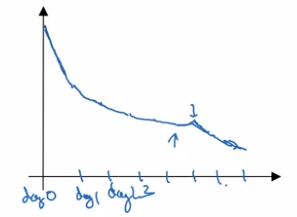• Caviar approach: train many models in parallel

Have enough computation power.
Different model/hyperparams being trained at the same time in parallel, pick the best one.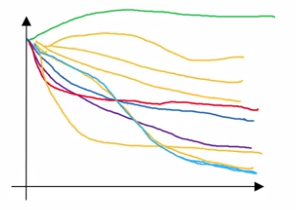## Batch Normalization

Batch normalization:
(in some cases) makes NN much more robust, and DNN much easier to train.

### Normalizing activations in a network

In pre-DL: normalize inputs to speedup learning. "make contours round"In NN: normalize the activation `a[l-1]` from previous layer could help (in practice, usually normalize `z[l-1]`.)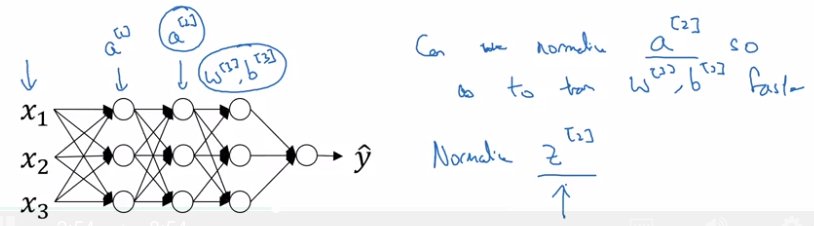BatchNorm algo:
intermediate values at each layer: `z[l]`
→ compute mean & variance
⇒ get normalized `z[l]_normed`. (mean=0, std=1)
trasform `z[l]_normed` to `z_tilde[l]` (mean=`beta`, std=`gamma`, beta and gamma are learnable params),
reason: for hidden units, want to move/stretch the support of hidden inputs, so as to profit from non-linearity of activation function.### Fitting Batch Norm into a neural network

Add batchnorm to NN: replace z[l] to z_tilde[l] at each layer before activation g[l].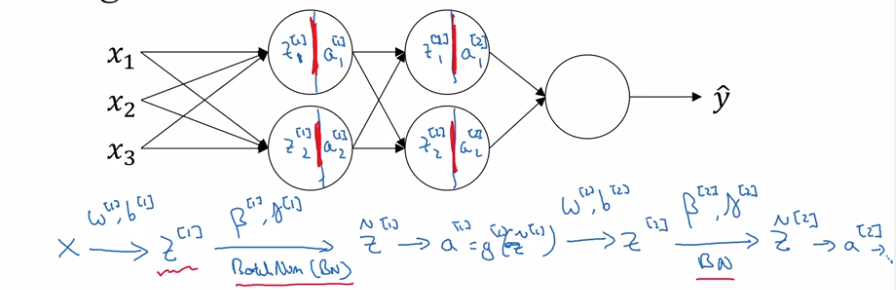Extra params to learn: `gamma[l]` and `beta[l]` at each layer.

In practice: no need to implement all details of BN, use DL framework.

No bias term (b) in BN:
z[l] = W[l] * a[l-1] + b[l]
but z[l] will be centered anyway → `b[l]` is not useful.
`b[l]` is replaced by `beta[l]`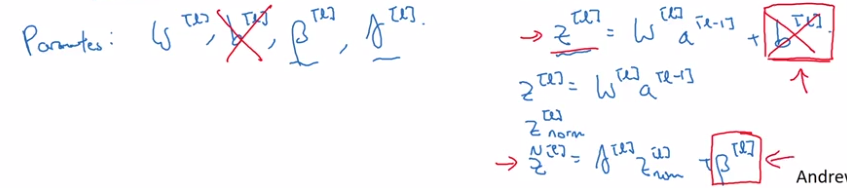Dimension of beta[l], gamma[l]: the same as b[l] ( = n[l] * 1).

### Why does Batch Norm work?

intuition 1: similar to normalizing input ("make contours round")

intuition 2: weights in deeper layers are more robust to changes in ealier layer weights.
i.e. Robost to data distribution changing. ("covariant shift")

motivating example:
cat-classification, trained all with black cats, but applied to colored cats.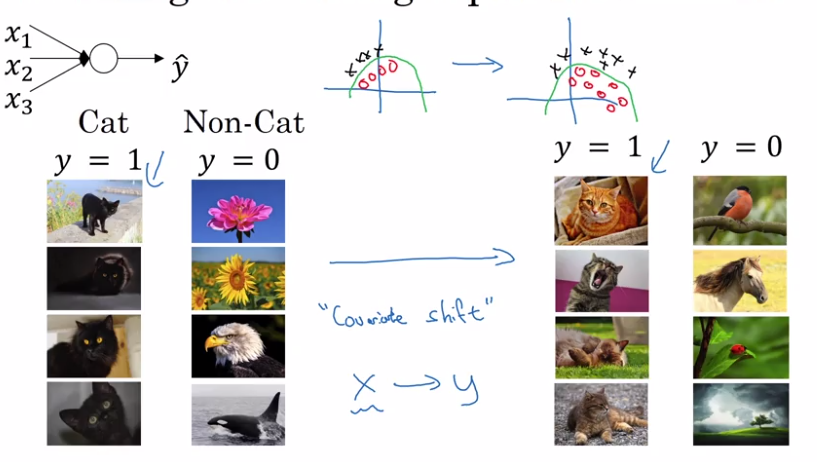For NN, consider the 3rd layer's units:
input features: a,
if cover the first 2 layers, this is a NN to map from a to y_hat
⇒ but when weights w,b are updated in GD, a's distribution is always changing.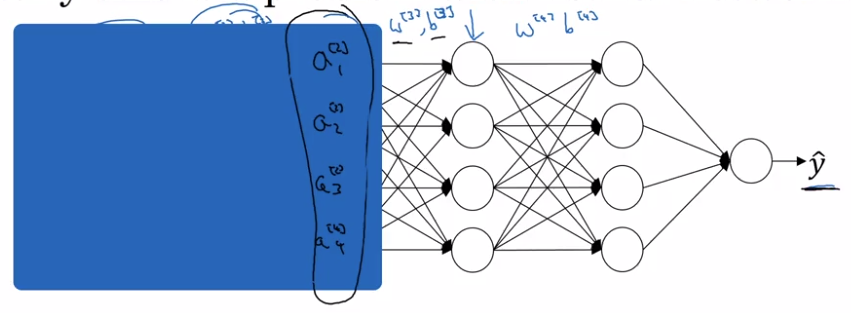intuition: With BN, a are ensured to always have the same mean/variance
→ "data distribution" is unchanged → later layers can learn more easily, independent of previous layer's weights' change.

intuition 2: BN as regularization
each minibatch is scaled by mean/var of just that minibatch
add noise to the transformation from z[l] to z_tilde[l].
⇒ similar to dropout, add noise to each layer's activations.
therefore BN have (slight) regularization effect (thie regularization effect gets smaller as minibatch size grows).
(This is an unintended side effect.)

### Batch Norm at test time

At training time, z[l] is standarlized over each minibatch.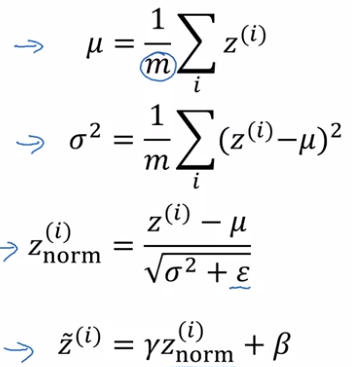⇒ But at test time needs to treat examples one at a time.
→ estimate the value of meu/sigma2
⇒ using exp-weighted-avg estimator across minibatchs (with beta close to 1 → ~running average).
at test time, just use the latest value of this exp-weighted-avg estimation as meu/sigma2.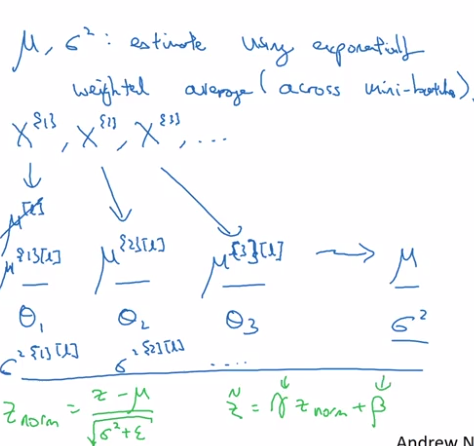## Multiclass classification

### Softmax Regression

So far: only binary classification
generalize logistic regression to >2 classes ⇒ softmax regression.

`C` = #classes, = #units in output layer
each component in y_hat is probability of one class, y_hat is normalized.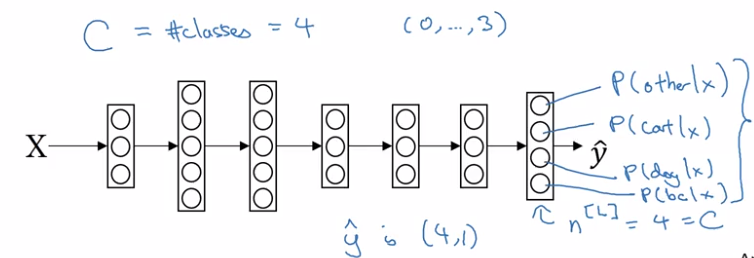softmax layer:

• z[L] = W[L] * a[l-1] + b[L] — same as before
• a[L] = y_hat = g(z[L])

activation function: softmax
take exp(z[L]) --element-wise, and then normalize: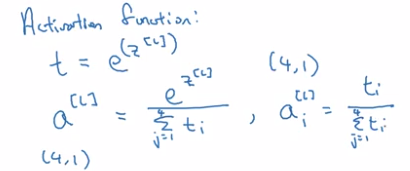The softmax activation function is unusual because it takes a vector instead of scalar.
Softmax is generalization of logistic regression: decision boundary of a single-layer (no hidden layer) softmax is also linear: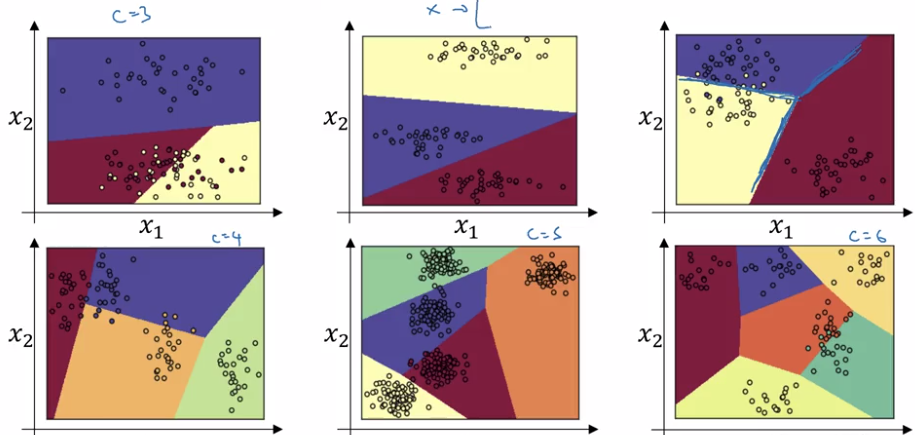### Training a softmax classifier

understanding softmax

• "softmax" is in contrast to "hardmax":

hardmax[i]= 1 if z_i=max else 0.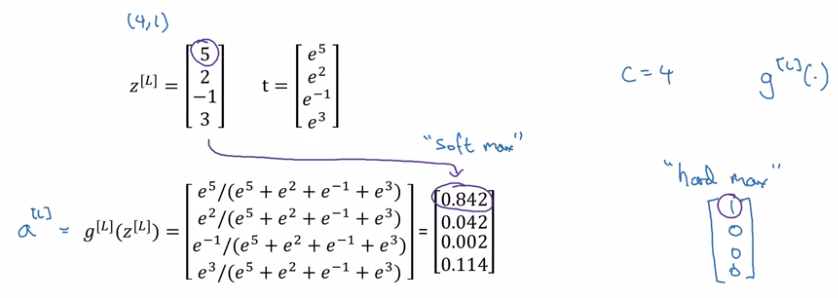• When C = 2, softmax reduces to logistic regression.

softmax(C=2) = [logistic-reg(), 1-logistic-reg]

loss function
recall: loss function in logistic regression
L(y, y_hat) = -1 * sum( y_i * log yhat_i + (1-y_i) * log(yhat_i) )
→ want y_hat big when y_i=1, small when y_i=0

• training label y: one-hot encoding.
• prediciton y_hat: probability vector

loss function:

• if y_k=1, want to make yhat_k big→ max-likelihood estimation.

GD with softmax
fwdprop:
Z[L] --(softmax)--> a[L]=y_hat → L(y_hat, y)
backprop:
dZ[L] = y_hat - y

## Introduction to programming frameworks

### Deep learning frameworks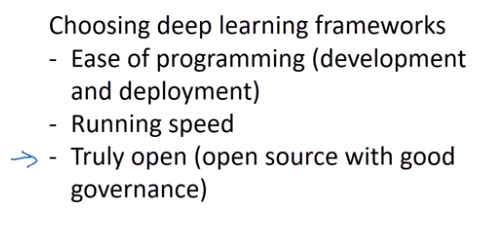### TensorFlow

motivating problem: minimize cost function `J(w) = (w-5)^2`

• `import tensorflow as tf`
• define parameter to optimize:
`w = tf.Variable(0, dtype=tf.float32)`

• define cost function:
```cost = tf.add(tf.add(w**2), tf.multiply(-10., w)), 25) # w^2 - 10w + 25 # also possible to use tf-reloaded operators: cost = w**2 - 10 * w + 25```

• tells tf to minimize the cost with GD optimizer:

`train = tf.train.GradientDescentOptimizer(0.01).minimize(cost)`
till now the computation graph is defined → backward derivatives are auto-computed.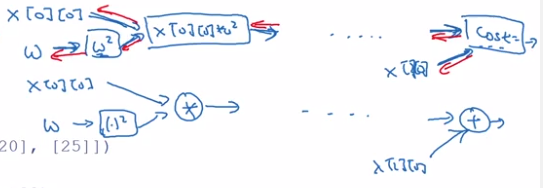• start the training

Quite idiomatic process:
initialize vars → create session → run operations in session

```init = tf.global_variables_initializer()
session = tf.Session()
session.run(init)
session.run(train) # run 1 iteration of training
```

alternative format:

```with tf.Session() as session:
session.run(init)
session.run(train)
```
• To inspect the value of a parameter: `print(session.run(w))`
• Run 1000 iters of GD:

```for i in range(1000): session.run(train)```

Let loss function depends on training data:

• define training data as placer holder.
a placerholder is a variable whose value will be assigned later.
```x = tf.placeholder(tf.float32, [3,1]) cost = x * w**2 + x * w + x```

• feed actual data value to placerholder: use feed_dict in session.run()

```data = np.array([1., -10., 25.]).reshape((3,1) session.run(train, feed_dict={x: data})```

#### Part 7 of series «Andrew Ng Deep Learning MOOC»：

comments powered by Disqus